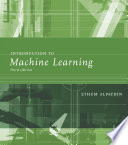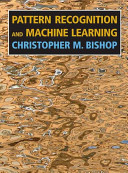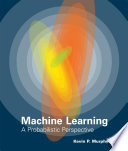### Machine Learning 1

Data is displayed for academic year: 2023./2024.

#### Course Description

Machine Learning is a branch of artificial intelligence concerned with the design of algorithms that improve their performance based on empirical data. It has become one of the most active and exciting areas of computer science research, in large part because of its wide-spread applicability, ranging from data mining and pattern recognition to robotics, computer vision, computational biology, and computational linguistics. This course gives an in-depth coverage of the theory and principles of machine learning, and gives an overview of machine learning applications. The course covers two main approaches to machine learning: supervised learning (classification and regression) and unsupervised learning (clustering and dimensionality reduction).

#### Study Programmes

[FER3-EN] Control Systems and Robotics - profile
(1. semester)
[FER3-EN] Data Science - profile
(1. semester)

#### Learning Outcomes

1. Define the basic concepts of machine learning
2. Distinguish between generative and discriminative, parametric and nonparametric and probabilistic and nonprobabilistic models models
3. Explain the theoretical assumptions, advantages, and disadvantages of basic machine learning algorithms
4. Apply model selection and statistical evaluation of the learned model
5. Apply various classification algorithms, inclusive generative, discriminative, and nonparametric ones
6. Apply clustering algorithms and cluster validation
7. Design and implement a machine learning method for classification/clustering and carry out its evalution
8. Assess the suitability of a machine learning algorithm for a given task

#### Forms of Teaching

Lectures

Lectures are given for 13 weeks in two two-hour sessions per week.

Exercises

Recitations are given for 13 weeks in one-hour sessions as the need arises.

Laboratory

Programming assignments, demonstrated to the instructor or teaching assistant.

#### Week by Week Schedule

1. Machine learning tasks and applications, Machine learning approaches and paradigms, Hypothesis, model, parameter space, version space, Inductive learning and inductive bias, language and preference bias, Loss function and error function, Overfitting and model selection, empirical and structural risk minimization
2. Least-squares regression, maximum likelihood estimation for linear regression, Ridge regression, Maximum entropy model, Feature mapping functions
3. Hypothesis, model, parameter space, version space, Perceptron (learning paradigms, Hebbian learning, competitive learning, Boltzmann learning)
4. Logistic regression, Generalized linear models (exponential family, ML and MAP estimation)
5. Support vector machine for classification.
6. Lazy classification (k-NN), Kernel functions (RBF, graph kernels, Mercer kernels, linear kernels), Kernel trick
7. Bagging and boosting, Stacked generalization
8. Midterm exam
9. Maximum likelihood estimator, Maximum aposteriori estimator, Laplace estimator, Beta-binomial model, Dirichlet-multinomial model
10. Bayes decision rule for classification, Naïve Bayes classifier, Multivariate Gaussian Bayes model
11. Bayesian networks, Exact inference (belief propagation, variable elimination, clique trees), Monte Carlo inference (rejection sampling, importance sampling)
12. Gaussian mixture model for clustering, K-means algorithm, K-medoids algorithm
13. Confusion matrix-based performance measures (accuracy, precision, recall, sensitivity, F-score), Multiclass performance measures, Receiver operating characteristic (ROC) analysis, Resampling error estimation (cross-validation, nested cross-validation, leave-one-out), Statistical testing of classifier performance, Debugging classifier algorithms
14. Feature selection (filter methods, subset selection, wrapper method)
15. Final exam

#### LiteratureEthem Alpaydin (2020.), Introduction to Machine Learning, MIT PressChristopher M. Bishop (2007.), Pattern Recognition and Machine Learning, SpringerKevin P. Murphy (2012.), Machine Learning, MIT Press

#### General

ID 223767
Winter semester
5 ECTS
L3 English Level
L1 e-Learning
45 Lectures
0 Seminar
15 Exercises
15 Laboratory exercises
0 Project laboratory